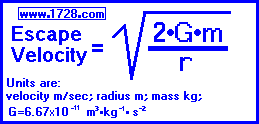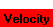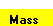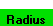Escape Velocity and Surface Gravity Ultra Calculator Scroll down for instructions.Solving for:  or  orDo not enter commas.
 I N S T R U C T I O N S This calculator can solve for escape velocity, or a gravitational body's mass, radius or surface gravity and will automatically calculate the value of 'g' - the gravitational constant. (For Earth, the value of 'g' is 9.80665 meters per second2.) You can input a great many different measurements: miles per second, kilometers per second, tons, kilograms, etc. When using this calculator, always choose what you are solving for first. Next, from the escape velocity, mass and radius rows, select the units you will be inputting. Enter the numbers and then click calculate.